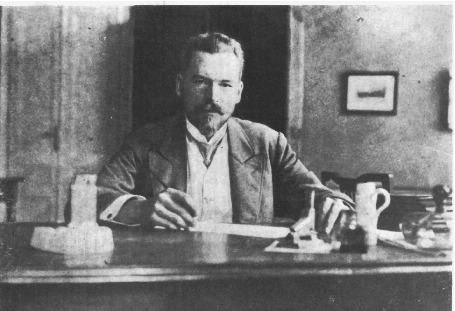#33rd M. Smoluchowski Symposium on Statistical Physics

3-4 December 2020
Europe/Warsaw timezone

## Temperature-dependent Smoluchowski equations

3 Dec 2020, 18:06
1m
poster

### Speaker

Prof. Nikolai Brilliantov (Skolkovo Institute of Science and Technology)

### Description

We report a new class of Smoluchowski-like equations for ballistically aggregating particles in a space-uniform system. They naturally emerge when the derivation of the aggregation equations is performed starting from the Boltzmann kinetic equation for the mass-velocity distribution functions . Then the system of equations for the zero-order moments of the distribution functions – the concentrations of aggregates of different size, corresponds to the conventional Smoluchowski equations. The aggregation rate coefficients of these equations depend on the second moments of the distribution function – partial temperatures of the aggregates. In this way, we obtain two coupled systems of equations – for the concentrations and temperatures . We show that, depending on the aggregation probability, these equations provide two main types of scaling. The first one, when all collisions are aggregative leads to Smoluchowski-type evolution with a gradual decrease in temperature. The second one, when only a small fraction of collisions are aggregative, results in a stunning effect of the aggregation with temperature growth. We show that the temperature increase may be stable and perpetual until the system remains large enough for the notion of the temperature to persist. We also estimate the system parameters (restitution coefficient, aggregation probability), which can lead to this type of scaling. The theoretical results are confirmed by the numerical simulations.

 N. Brilliantov, T. Poeschel, and A. Formella, Increasing temperature of cooling granular gases, Nature Communications, 9 (2018) 797.​

### Primary author

Prof. Nikolai Brilliantov (Skolkovo Institute of Science and Technology)

### Co-authors

Mr. Alexander Osinsky (Skolkovo Institute of Science and Technology) Prof. Arno Formella (Department of Computer Science, University of Vigo) Prof. Thorsten Poeschel (Institute for Multiscale Simulation, Friedrich-Alexander-Universität Erlangen-Nürnberg)

### Presentation Materials

There are no materials yet.
###### Your browser is out of date!

Update your browser to view this website correctly. Update my browser now

×